# Volume Of A Cylinder Worksheet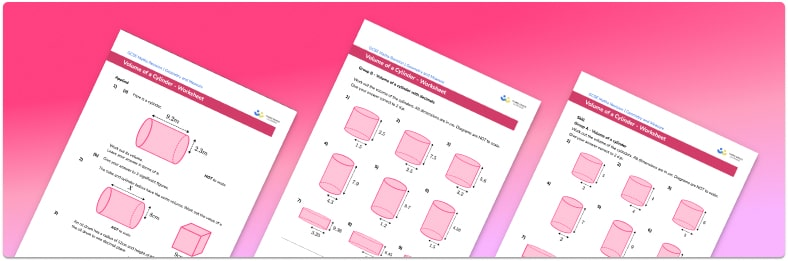• Section 1 of the volume of a cylinder worksheet contains 27 skills-based volume of a cylinder questions, in 3 groups to support differentiation
• Section 2 contains 4 applied volume of a cylinder questions with a mix of word problems and deeper problem solving questions
• Section 3 contains 3 foundation and higher level GCSE exam style volume of a cylinder questions
• An answer key and a mark scheme for all volume of a cylinder questions are provided
• Questions follow variation theory with plenty of opportunities for students to work independently at their own level
• All questions created by fully qualified expert secondary maths teachers
• Suitable for GCSE maths revision for AQA, OCR and Edexcel exam boards

• This field is for validation purposes and should be left unchanged.

You can unsubscribe at any time (each email we send will contain an easy way to unsubscribe). To find out more about how we use your data, see our privacy policy.

### Volume of a cylinder at a glance

Volume is a measure of how much space there is inside a 3D shape. To calculate the volume of a cylinder we use the formula V=π(r^2)h where r is the radius of the base of the cylinder and h is the height of the cylinder. Notice that this is very similar to the formula for the volume of a prism, V = \text{Cross-sectional area} \times \text{Height} . In the case of a cylinder the cross-section is a circle, with the area of a circle being π(r^2) and this is multiplied by the height or length of the shape.

The volume of the cylinder is measured in units cubed, for example cm^3 or mm^3 . We will often be asked to either give an answer in terms of pi ( π ) or to round the answer to a given degree of accuracy, such as to the nearest tenth.

Looking forward, students can then progress to additional volume worksheets and other geometry worksheets, for example a simplifying expressions worksheet or simultaneous equations worksheet.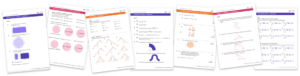For more teaching and learning support on Geometry our GCSE maths lessons provide step by step support for all GCSE maths concepts.

## Related worksheets

Volume Of A Cone Worksheet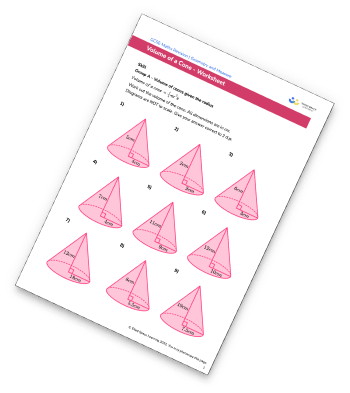Volume Of A Cuboid Worksheet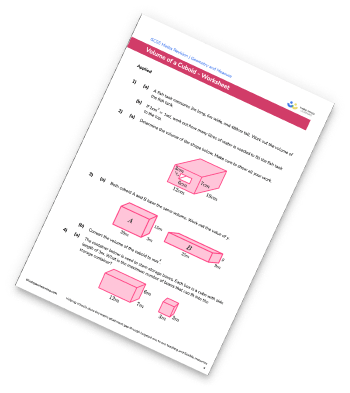Congruent Triangles Worksheet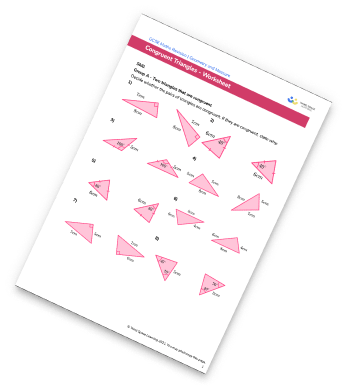Surface Area Of A Triangular Prism Worksheet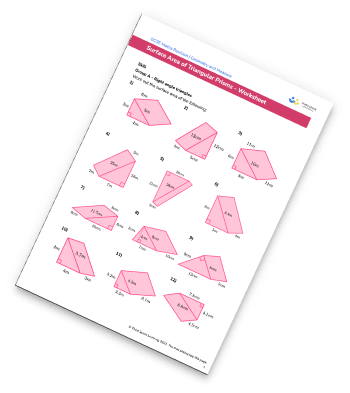## Do you have KS4 students who need more focused attention to succeed at GCSE?There will be students in your class who require individual attention to help them succeed in their maths GCSEs. In a class of 30, it’s not always easy to provide.

Help your students feel confident with exam-style questions and the strategies they’ll need to answer them correctly with our dedicated GCSE maths revision programme.

Lessons are selected to provide support where each student needs it most, and specially-trained GCSE maths tutors adapt the pitch and pace of each lesson. This ensures a personalised revision programme that raises grades and boosts confidence.

Find out more